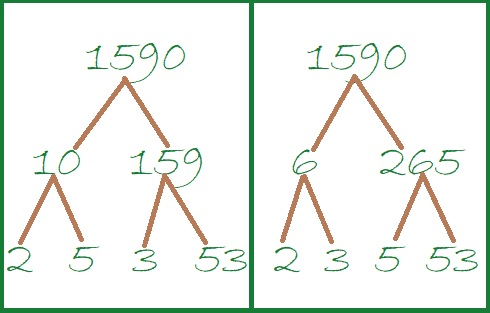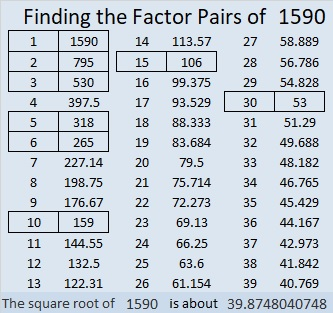# 1590 A Single Rose

### Today’s Puzzle:

A single rose can be an elegant expression of affection. This single rose is a level 5 puzzle. Can you find its factors?Here’s the same puzzle without any added color:### Two Factor Trees for 1590:

There are several possible factor trees for 1590. Here are two of them.### Factors of 1590:

• 1590 is a composite number.
• Prime factorization: 1590 = 2 × 3 × 5 × 53
• 1590 has no exponents greater than 1 in its prime factorization, so √1590 cannot be simplified.
• The exponents in the prime factorization are 1, 1, 1, and 1. Adding one to each exponent and multiplying we get (1 + 1)(1 + 1)(1 + 1)(1 + 1) = 2 × 2 × 2 × 2 = 16. Therefore 1590 has exactly 16 factors.
• The factors of 1590 are outlined with their factor pair partners in the graphic below.### More about the Number 1590:

1590 is the hypotenuse of FOUR Pythagorean triples:
138-1584-1590, which is 6 times (23-264-265),
576-1482-1590, which is 6 times (96-247-265)
840-1350-1590, which is 30 times (28-45-53),
954-1272-1590, which is (3-4-5) times 318.